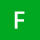Home Communities
IT Knowledge
Inspiration
Languages
EN

# Python - replace regex with regex using re.sub()

0 pointsFaith-W
503

How can I replace a pattern (regex) with another regex using `re.sub()` on a string in Python?

I want to convert camel case to sentence case so I need to replace every capital letter with the same letter in lower case followed by a space character.

Example input:

``mySampleText``

What I want to receive:

``My sample text``

I tried this but it doesn't work:

``````import re

def sentence_case(string):
if string != "":
result = re.sub("([A-Z])", " \$1", string)       # <---- this doesn't work
return result[:1].upper() + result[1:].lower()
return

text = "mySampleText"
print(sentence_case(text))``````

Output:

``My \$1ample \$1ext``
0 pointsFaith-W
503

The `r` specifier was the issue.

It worked when I used the `r` specifier (raw string) for regular expression like this:

``````import re

def sentence_case(string):
if string != "":
result = re.sub("([A-Z])", r" \1", string)
return result[:1].upper() + result[1:].lower()
return

text = "mySampleText"
print(sentence_case(text))``````

Output:

``My sample text``